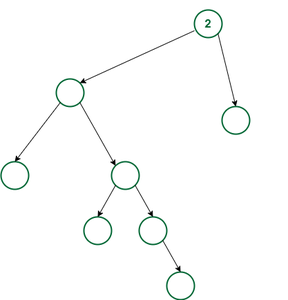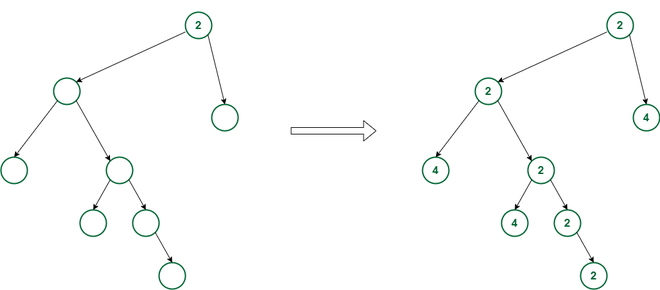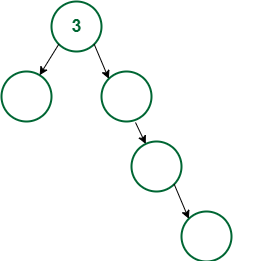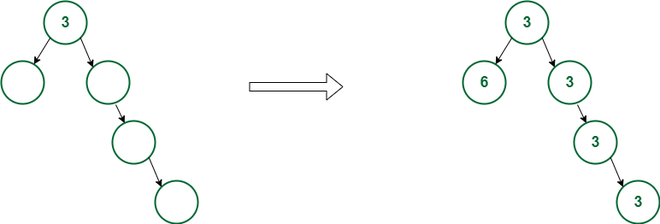Related Articles

# Minimize sum of node values by filling given empty Tree such that each node is GCD of its children

• Difficulty Level : Hard
• Last Updated : 23 Sep, 2021

Given a Binary Tree consisting of N nodes having no values in it and an integer X, that represents the value of the root node, the task is to find the minimum sum of all the nodes value of the given Tree such that the value of each node must be the value of GCDs of its children. Also, no two siblings can have the same value.

Examples:

Input:Output: 22
Explanation: The given tree can be filled as shown below:Input:Output: 18
Explanation: The given tree can be filled as shown below:Approach: In order to minimize the sum, both of the children can have the value of X and 2*X where X is the value of the parent. Now, if the node has only one child, then its value is going to be equal to its parent node. But to decide which child should have a value of X and 2*X to get the minimum sum, the depth of each subtree for every node will be considered. The child having more depth will be given a value of X so that it can transfer it to more of its children while another one will get the value of 2*X. Follow the below steps to solve this problem:

1. Find the depth of each node and store it in a map alongside the node address as the key.
2. Now, perform the DFS Traversal, starting from the root node, and in each call assign the node a value of X if it has more depth than its sibling. Otherwise, assign the value 2*X.
3. After the above step, find the sum of both left and right child values while backtracking and return the total sum, i.e., the sum of the value of the left child, right child, and current node in each call.
4. After completing the above steps, print the value returned from the DFS Call as the minimum sum possible.

Below is the implementation of the above approach:

## C++

 `// C++ program for the above approach``#include ``using` `namespace` `std;` `// Structure of Tree Node``class` `Node {``public``:``    ``int` `val;``    ``Node *left, *right;``    ``Node(``int` `val)``    ``{``        ``this``->val = val;``        ``left = NULL;``        ``right = NULL;``    ``}``};` `class` `Tree {``public``:``    ``unordered_map depth;` `    ``// Function to find the depth of all``    ``// nodes and store it in map depth``    ``int` `findDepth(Node* cur)``    ``{``        ``int` `mx = 0;``        ``if` `(cur->left) {``            ``mx = findDepth(cur->left);``        ``}``        ``if` `(cur->right) {``            ``mx = max(mx, findDepth(cur->right));``        ``}` `        ``// Update and return the maximum``        ``// depth of the tree``        ``return` `depth[cur] = mx + 1;``    ``}` `    ``// Function to assign values to nodes``    ``// and return the minimum sum possible``    ``int` `dfs(Node* cur, ``bool` `flag, ``int` `parValue)``    ``{``        ``if` `(parValue != -1) {``            ``if` `(flag)``                ``cur->val = parValue;``            ``else``                ``cur->val = parValue * 2;``        ``}``        ``int` `l = 0, r = 0;``        ``if` `(cur->left && cur->right) {``            ``if` `(depth[cur->left] > depth[cur->right]) {``                ``l = dfs(cur->left, 1, cur->val);``                ``r = dfs(cur->right, 0, cur->val);``            ``}``            ``else` `{``                ``l = dfs(cur->left, 0, cur->val);``                ``r = dfs(cur->right, 1, cur->val);``            ``}``        ``}``        ``else` `if` `(cur->left) {``            ``l = dfs(cur->left, 1, cur->val);``        ``}``        ``else` `if` `(cur->right) {``            ``r = dfs(cur->right, 1, cur->val);``        ``}` `        ``return` `l + r + cur->val;``    ``}` `    ``// Function to find the minimum sum``    ``// for the given tree by assign the``    ``// values to the node according to``    ``// the given criteria``    ``int` `minimumSum(Node* root)``    ``{``        ``// Find the maximum depth``        ``findDepth(root);` `        ``// Calculate the minimum sum and``        ``// return it``        ``return` `dfs(root, 1, -1);``    ``}``};` `// Driver Code``int` `main()``{` `    ``// Given root node value``    ``int` `X = 2;` `    ``// Given empty tree structure``    ``Node* root = ``new` `Node(X);``    ``root->left = ``new` `Node(-1);``    ``root->right = ``new` `Node(-1);``    ``root->left->left = ``new` `Node(-1);``    ``root->left->right = ``new` `Node(-1);``    ``root->left->right->left = ``new` `Node(-1);``    ``root->left->right->right = ``new` `Node(-1);``    ``root->left->right->right->left = ``new` `Node(-1);` `    ``Tree t;` `    ``// Fill the tree and print minimum tree sum``    ``cout << t.minimumSum(root);` `    ``return` `0;``}`

## Java

 `// Java program for the above approach``import` `java.util.*;``public` `class` `Main``{``    ``// Structure of Tree Node``    ``static` `class` `Node {``        ` `        ``public` `int` `val;``        ``public` `Node left, right;``        ` `        ``public` `Node(``int` `val)``        ``{``            ``this``.val = val;``            ``left = right = ``null``;``        ``}``    ``}``    ` `    ``static` `HashMap depth = ``new` `HashMap<>();``    ` `    ``// Function to find the depth of all``    ``// nodes and store it in map depth``    ``static` `int` `findDepth(Node cur)``    ``{``        ``int` `mx = ``0``;``        ``if` `(cur.left != ``null``) {``            ``mx = findDepth(cur.left);``        ``}``        ``if` `(cur.right != ``null``) {``            ``mx = Math.max(mx, findDepth(cur.right));``        ``}``    ` `        ``// Update and return the maximum``        ``// depth of the tree``        ``depth.put(cur, mx + ``1``);``        ``return` `depth.get(cur);``    ``}``    ` `    ``// Function to assign values to nodes``    ``// and return the minimum sum possible``    ``static` `int` `dfs(Node cur, ``int` `flag, ``int` `parValue)``    ``{``        ``if` `(parValue != -``1``) {``            ``if` `(flag == ``1``)``                ``cur.val = parValue;``            ``else``                ``cur.val = parValue * ``2``;``        ``}``        ``int` `l = ``0``, r = ``0``;``        ``if` `(cur.left != ``null` `&& cur.right != ``null``) {``            ``if` `(depth.containsKey(cur.left) && depth.containsKey(cur.right) && depth.get(cur.left) > depth.get(cur.right)) {``                ``l = dfs(cur.left, ``1``, cur.val);``                ``r = dfs(cur.right, ``0``, cur.val);``            ``}``            ``else` `{``                ``l = dfs(cur.left, ``0``, cur.val);``                ``r = dfs(cur.right, ``1``, cur.val);``            ``}``        ``}``        ``else` `if` `(cur.left != ``null``) {``            ``l = dfs(cur.left, ``1``, cur.val);``        ``}``        ``else` `if` `(cur.right != ``null``) {``            ``r = dfs(cur.right, ``1``, cur.val);``        ``}``    ` `        ``return` `(l + r + cur.val);``    ``}``    ` `    ``// Function to find the minimum sum``    ``// for the given tree by assign the``    ``// values to the node according to``    ``// the given criteria``    ``static` `int` `minimumSum(Node root)``    ``{``        ``// Find the maximum depth``        ``findDepth(root);``    ` `        ``// Calculate the minimum sum and``        ``// return it``        ``return` `dfs(root, ``1``, -``1``);``    ``}``    ` `  ``// Driver code``    ``public` `static` `void` `main(String[] args)``    ``{``      ` `        ``// Given root node value``        ``int` `X = ``2``;``        ` `        ``// Given empty tree structure``        ``Node root = ``new` `Node(X);``        ``root.left = ``new` `Node(-``1``);``        ``root.right = ``new` `Node(-``1``);``        ``root.left.left = ``new` `Node(-``1``);``        ``root.left.right = ``new` `Node(-``1``);``        ``root.left.right.left = ``new` `Node(-``1``);``        ``root.left.right.right = ``new` `Node(-``1``);``        ``root.left.right.right.left = ``new` `Node(-``1``);``        ` `        ``// Fill the tree and print minimum tree sum``        ``System.out.print(minimumSum(root));``    ``}``}` `// This code is contributed by suresh07.`

## Python3

 `# Python3 program for the above approach` `# Structure of Tree Node``class` `Node:``    ``def` `__init__(``self``, val):``        ``self``.val ``=` `val``        ``self``.left ``=` `None``        ``self``.right ``=` `None` `depth ``=` `{}``   ` `# Function to find the depth of all``# nodes and store it in map depth``def` `findDepth(cur):``    ``mx ``=` `0``    ``if` `(cur.left !``=` `None``):``        ``mx ``=` `findDepth(cur.left)``    ``if` `(cur.right !``=` `None``):``        ``mx ``=` `max``(mx, findDepth(cur.right))` `    ``# Update and return the maximum``    ``# depth of the tree``    ``depth[cur] ``=` `mx ``+` `1``    ``return` `depth[cur]` `# Function to assign values to nodes``# and return the minimum sum possible``def` `dfs(cur, flag, parValue):``    ``if` `(parValue !``=` `-``1``):``        ``if` `flag:``            ``cur.val ``=` `parValue``        ``else``:``            ``cur.val ``=` `parValue ``*` `2``    ``l, r ``=` `0``,  ``0``;``    ``if` `(cur.left !``=` `None` `and` `cur.right !``=` `None``):``        ``if` `((cur.left ``in` `depth) ``and` `(cur.right ``in` `depth) ``and` `depth[cur.left] > depth[cur.right]):``            ``l ``=` `dfs(cur.left, ``1``, cur.val)``            ``r ``=` `dfs(cur.right, ``0``, cur.val)``        ``else``:``            ``l ``=` `dfs(cur.left, ``0``, cur.val)``            ``r ``=` `dfs(cur.right, ``1``, cur.val)``    ``elif` `(cur.left !``=` `None``):``        ``l ``=` `dfs(cur.left, ``1``, cur.val)``    ``elif` `(cur.right !``=` `None``):``        ``r ``=` `dfs(cur.right, ``1``, cur.val)` `    ``return` `(l ``+` `r ``+` `cur.val)` `# Function to find the minimum sum``# for the given tree by assign the``# values to the node according to``# the given criteria``def` `minimumSum(root):``  ` `    ``# Find the maximum depth``    ``findDepth(root)` `    ``# Calculate the minimum sum and``    ``# return it``    ``return` `dfs(root, ``1``, ``-``1``)` `# Given root node value``X ``=` `2` `# Given empty tree structure``root ``=` `Node(X)``root.left ``=` `Node(``-``1``)``root.right ``=` `Node(``-``1``)``root.left.left ``=` `Node(``-``1``)``root.left.right ``=``Node(``-``1``)``root.left.right.left ``=` `Node(``-``1``)``root.left.right.right ``=` `Node(``-``1``)``root.left.right.right.left ``=` `Node(``-``1``);` `# Fill the tree and print minimum tree sum``print``(minimumSum(root))` `# This code is contributed by mukesh07.`

## C#

 `// C# program for the above approach``using` `System;``using` `System.Collections.Generic;``class` `GFG {``    ` `    ``// Structure of Tree Node``    ``class` `Node {``       ` `        ``public` `int` `val;``        ``public` `Node left, right;``       ` `        ``public` `Node(``int` `val)``        ``{``            ``this``.val = val;``            ``left = right = ``null``;``        ``}``    ``}``    ` `    ``static` `Dictionary depth = ``new` `Dictionary();``   ` `    ``// Function to find the depth of all``    ``// nodes and store it in map depth``    ``static` `int` `findDepth(Node cur)``    ``{``        ``int` `mx = 0;``        ``if` `(cur.left != ``null``) {``            ``mx = findDepth(cur.left);``        ``}``        ``if` `(cur.right != ``null``) {``            ``mx = Math.Max(mx, findDepth(cur.right));``        ``}``   ` `        ``// Update and return the maximum``        ``// depth of the tree``        ``depth[cur] = mx + 1;``        ``return` `depth[cur];``    ``}``   ` `    ``// Function to assign values to nodes``    ``// and return the minimum sum possible``    ``static` `int` `dfs(Node cur, ``int` `flag, ``int` `parValue)``    ``{``        ``if` `(parValue != -1) {``            ``if` `(flag == 1)``                ``cur.val = parValue;``            ``else``                ``cur.val = parValue * 2;``        ``}``        ``int` `l = 0, r = 0;``        ``if` `(cur.left != ``null` `&& cur.right != ``null``) {``            ``if` `(depth.ContainsKey(cur.left) && depth.ContainsKey(cur.right) && depth[cur.left] > depth[cur.right]) {``                ``l = dfs(cur.left, 1, cur.val);``                ``r = dfs(cur.right, 0, cur.val);``            ``}``            ``else` `{``                ``l = dfs(cur.left, 0, cur.val);``                ``r = dfs(cur.right, 1, cur.val);``            ``}``        ``}``        ``else` `if` `(cur.left != ``null``) {``            ``l = dfs(cur.left, 1, cur.val);``        ``}``        ``else` `if` `(cur.right != ``null``) {``            ``r = dfs(cur.right, 1, cur.val);``        ``}``   ` `        ``return` `(l + r + cur.val);``    ``}``   ` `    ``// Function to find the minimum sum``    ``// for the given tree by assign the``    ``// values to the node according to``    ``// the given criteria``    ``static` `int` `minimumSum(Node root)``    ``{``        ``// Find the maximum depth``        ``findDepth(root);``   ` `        ``// Calculate the minimum sum and``        ``// return it``        ``return` `dfs(root, 1, -1);``    ``}``    ` `  ``static` `void` `Main() {``    ``// Given root node value``    ``int` `X = 2;``   ` `    ``// Given empty tree structure``    ``Node root = ``new` `Node(X);``    ``root.left = ``new` `Node(-1);``    ``root.right = ``new` `Node(-1);``    ``root.left.left = ``new` `Node(-1);``    ``root.left.right = ``new` `Node(-1);``    ``root.left.right.left = ``new` `Node(-1);``    ``root.left.right.right = ``new` `Node(-1);``    ``root.left.right.right.left = ``new` `Node(-1);``   ` `    ``// Fill the tree and print minimum tree sum``    ``Console.Write(minimumSum(root));``  ``}``}` `// This code is contributed by divyesh072019.`

## Javascript

 ``

Output
`22`

Time Complexity: O(N)
Auxiliary Space: O(1)

Attention reader! Don’t stop learning now. Get hold of all the important DSA concepts with the DSA Self Paced Course at a student-friendly price and become industry ready.  To complete your preparation from learning a language to DS Algo and many more,  please refer Complete Interview Preparation Course.

In case you wish to attend live classes with experts, please refer DSA Live Classes for Working Professionals and Competitive Programming Live for Students.

My Personal Notes arrow_drop_up